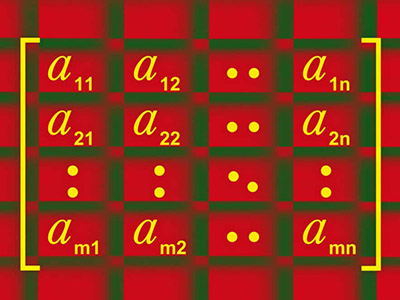ΓραμματείαSecretariat: 2410 684574 | ΦοιτητικάStudents: 2410 684387 g-ds@uth.gr
Select Page

# Linear Algebra

Υ306

3

#### Hours/Week - ECTS

4 – 5### Learning Outcomes

The main objective of the course is the complete understanding of the fundamental concepts of Linear Algebra which are necessary for the continuation of the studies. A partial goal of the course is the acquisition of specific technical knowledge (like for example: how can I solve a linear system? How can I calculate its eigenvalues?) which by themselves will be completely useless.

Specifically, students will be able to:

• Demonstrate their ability to understand and use the basic ideas of linear algebra, including the concepts of linear independence, linear transformations, bases and dimensions of vector spaces, eigenvalues, eigenvectors, and diagonalization.
• They compose clear and precise proofs, using the concepts of the lesson.

In addition, they will be able to:

• Determine whether a system of equations has a solution and find its general solution.
• Parse a table into a product of simpler tables.
• Solve systems of the form Ax = b , where A is an mxn matrix and x is a vector of !^n • Determine whether the columns of a given matrix are linearly dependent or not.
• Understand what the linear transformation is defined by x → Ax .
• Recognize various categories of special tables.
• Calculate the determinant of a given matrix.
• Identify the four fundamental subspaces of a given matrix and find their bases and deduce the existence and uniqueness of the solution. Define a subspace from a vector space.
• Change the coordinates of a vector from the basis to a standard basis
• Calculate the solution of least squares problems.
• Define the characteristic polynomial of a given array.
• Calculate the eigenvalues ​​and eigenvectors of a matrix.

### Indicative Module Content

• Determine if a system of equations has a solution and find its general solution.
• Analyze a table into a product of simpler tables.
• Solve systems of the form Ax = b, where A is an array/matrix mxn and x is a vector of Rn
• Determine whether the columns of a given table are linearly dependent or not.
• Understand what is the linear transformation defined by x → Ax.
• Recognize different categories of special tables.
• Calculate the determinant of a given table.
• Identify the four fundamental subspaces of a given table and find their bases and conclude the existence and uniqueness of the solution.
• Define a subspace from a vector space.
• Change the coordinates of a vector from the base to a standard base.
• Calculate the solution of least squares problems.
• Define the characteristic polynomial of a given array.
• Calculate the eigenvalues ​​and eigenvectors of a table.